1575901604

# Implementing a Recurrent Neural Network from Scratch in Python

The main objective of this post is to implement an RNN from scratch and provide an easy explanation as well to make it useful for the readers. Implementing any neural network from scratch at least once is a valuable exercise. It helps you gain an understanding of how neural networks work and here we are implementing an RNN which has its own complexity and thus provides us with a good opportunity to hone our skills.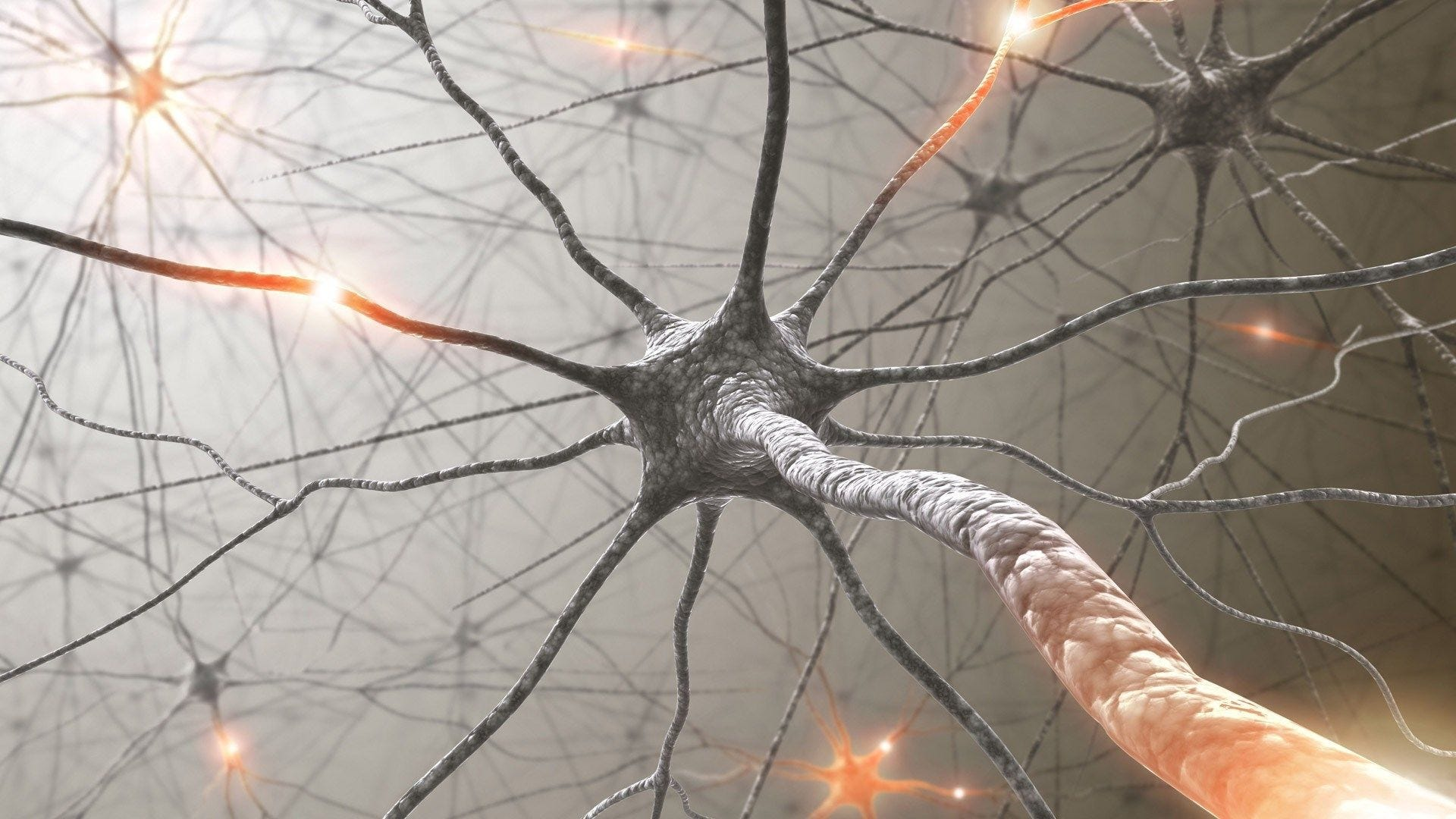Source

There are various tutorials that provide a very detailed information of the internals of an RNN. You can find some of the very useful references at the end of this post. I could understand the working of an RNN rather quickly but what troubled me most was going through the BPTT calculations and its implementation. I had to spent some time to understand and finally put it all together. Without wasting any more time, let us quickly go through the basics of an RNN first.

## What is an RNN?

A recurrent neural network is a neural network that is specialized for processing a sequence of data `x(t)= x(1), . . . , x(τ)` with the time step index `t` ranging from `1 to τ`. For tasks that involve sequential inputs, such as speech and language, it is often better to use RNNs. In a NLP problem, if you want to predict the next word in a sentence it is important to know the words before it. RNNs are called recurrent because they perform the same task for every element of a sequence, with the output being depended on the previous computations. Another way to think about RNNs is that they have a “memory” which captures information about what has been calculated so far.

Architecture : Let us briefly go through a basic RNN network.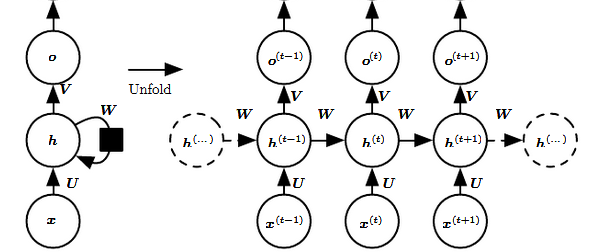Reference

The left side of the above diagram shows a notation of an RNN and on the right side an RNN being unrolled (or unfolded) into a full network. By unrolling we mean that we write out the network for the complete sequence. For example, if the sequence we care about is a sentence of 3 words, the network would be unrolled into a 3-layer neural network, one layer for each word.

Input: `x(t)`​ is taken as the input to the network at time step `t`. For example, `x1`, could be a one-hot vector corresponding to a word of a sentence.

Hidden state: `h(t)`​ represents a hidden state at time t and acts as “memory” of the network. `h(t)`​ is calculated based on the current input and the previous time step’s hidden state: `h(t)​ = f(U x(t)​ + W h(t−1)​).` The function `f` is taken to be a non-linear transformation such as tanh, ReLU.

Weights: The RNN has input to hidden connections parameterized by a weight matrix U, hidden-to-hidden recurrent connections parameterized by a weight matrix W, and hidden-to-output connections parameterized by a weight matrix V and all these weights (U,V,W) are shared across time.

Output: `o(t)`​ illustrates the output of the network. In the figure I just put an arrow after `o(t)` which is also often subjected to non-linearity, especially when the network contains further layers downstream.

## Forward Pass

The ﬁgure does not specify the choice of activation function for the hidden units. Before we proceed we make few assumptions: 1) we assume the hyperbolic tangent activation function for hidden layer. 2) We assume that the output is discrete, as if the RNN is used to predict words or characters. A natural way to represent discrete variables is to regard the output `o` as giving the un-normalized log probabilities of each possible value of the discrete variable. We can then apply the softmax operation as a post-processing step to obtain a vector `ŷ`of normalized probabilities over the output.

The RNN forward pass can thus be represented by below set of equations.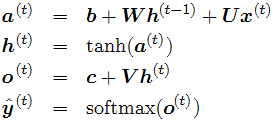This is an example of a recurrent network that maps an input sequence to an output sequence of the same length. The total loss for a given sequence of `x` values paired with a sequence of `y` values would then be just the sum of the losses over all the time steps. We assume that the outputs `o(t)`are used as the argument to the softmax function to obtain the vector `ŷ` of probabilities over the output. We also assume that the loss `L` is the negative log-likelihood of the true target `y(t)`given the input so far.

## Backward Pass

The gradient computation involves performing a forward propagation pass moving left to right through the graph shown above followed by a backward propagation pass moving right to left through the graph. The runtime is O(τ) and cannot be reduced by parallelization because the forward propagation graph is inherently sequential; each time step may be computed only after the previous one. States computed in the forward pass must be stored until they are reused during the backward pass, so the memory cost is also O(τ). The back-propagation algorithm applied to the unrolled graph with O(τ) cost is called back-propagation through time (BPTT). Because the parameters are shared by all time steps in the network, the gradient at each output depends not only on the calculations of the current time step, but also the previous time steps.

Given our loss function L, we need to calculate the gradients for our three weight matrices U, V, W, and bias terms b, c and update themwith a learning rate `α`. Similar to normal back-propagation, the gradient gives us a sense of how the loss is changing with respect to each weight parameter. We update the weights W to minimize loss with the following equation: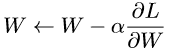The same is to be done for the other weights U, V, b, c as well.

Let us now compute the gradients by BPTT for the RNN equations above. The nodes of our computational graph include the parameters U, V, W, b and c as well as the sequence of nodes indexed by t for x (t), h(t), o(t) and L(t). For each node `n` we need to compute the gradient `∇nL` recursively, based on the gradient computed at nodes that follow it in the graph.

Gradient with respect to output o(t) is calculated assuming the o(t) are used as the argument to the softmax function to obtain the vector `ŷ` of probabilities over the output. We also assume that the loss is the negative log-likelihood of the true target y(t).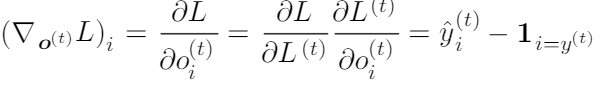Please refer here for deriving the above elegant solution.

Let us now understand how the gradient flows through hidden state h(t). This we can clearly see from the below diagram that at time t, hidden state h(t) has gradient flowing from both current output and the next hidden state.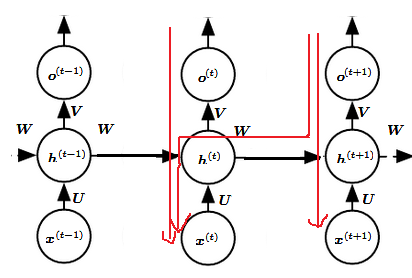Red arrow shows gradient flow

We work our way backward, starting from the end of the sequence. At the ﬁnal time step τ, h(τ) only has o(τ) as a descendant, so its gradient is simple: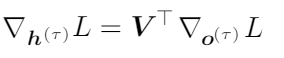We can then iterate backward in time to back-propagate gradients through time, from t=τ −1 down to t = 1, noting that h(t) (for t < τ ) has as descendants both o(t) and h(t+1). Its gradient is thus given by: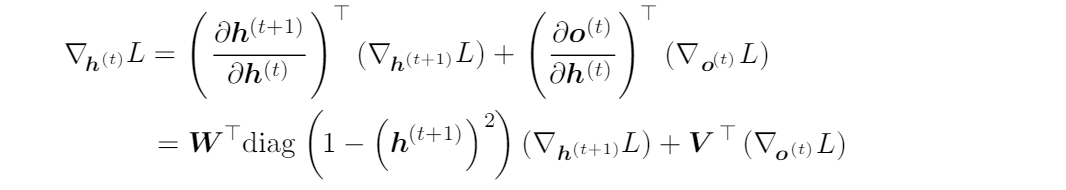Once the gradients on the internal nodes of the computational graph are obtained, we can obtain the gradients on the parameter nodes. The gradient calculations using the chain rule for all parameters is: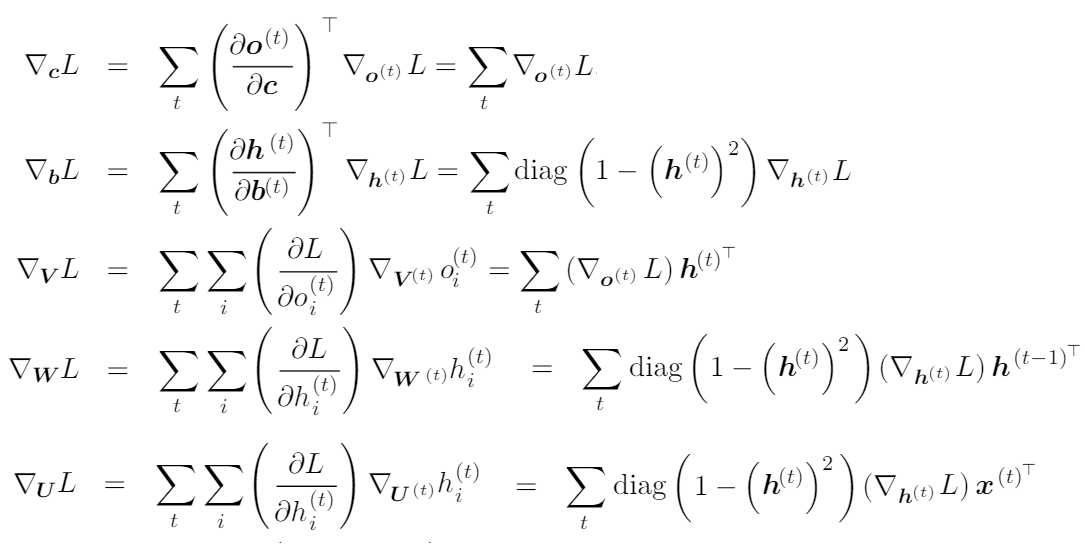We are not interested to derive these equations here, rather implementing these. There are very good posts here and here providing detailed derivation of these equations.

## Implementation

We will implement a full Recurrent Neural Network from scratch using Python. We will try to build a text generation model using an RNN. We train our model to predict the probability of a character given the preceding characters. It’s a generative model. Given an existing sequence of characters we sample a next character from the predicted probabilities, and repeat the process until we have a full sentence. This implementation is from Andrej Karparthy great post building a character level RNN. Here we will discuss the implementation details step by step.

General steps to follow:

1. Initialize weight matrices U, V, W from random distributionand bias b, c with zeros
2. Forward propagation to compute predictions
3. Compute the loss
4. Back-propagation to compute gradients
5. Update weights based on gradients
6. Repeat steps 2–5

## Step 1: Initialize

To start with the implementation of the basic RNN cell, we first define the dimensions of the various parameters U,V,W,b,c.

Dimensions:Let’s assume we pick a vocabulary size `vocab_size= 8000` and a hidden layer size `hidden_size=100`. Then we have: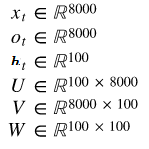Vocabulary size can be the number of unique chars for a char based model or number of unique words for a word based model.

With our few hyper-parameters and other model parameters, let us start defining our RNN cell.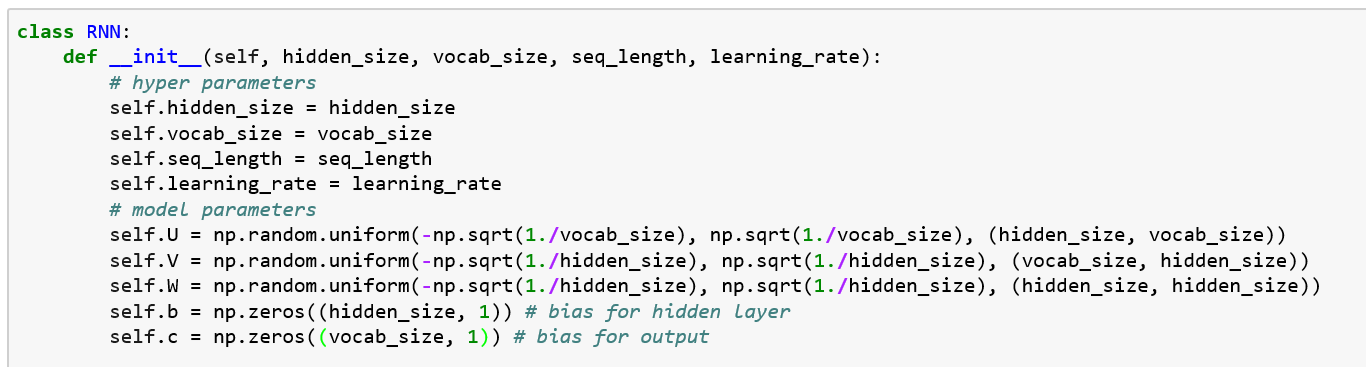Proper initialization of weights seems to have an impact on training results there has been lot of research in this area. It turns out that the best initialization depends on the activation function (tanh in our case) and one recommended approach is to initialize the weights randomly in the interval from`[ -1/sqrt(n), 1/sqrt(n)]`where `n` is the number of incoming connections from the previous layer.

## Step 2: Forward pass

Straightforward as per our equations for each timestamp t, we calculate hidden state hs[t] and output os[t] applying softmax to get the probability for the next character.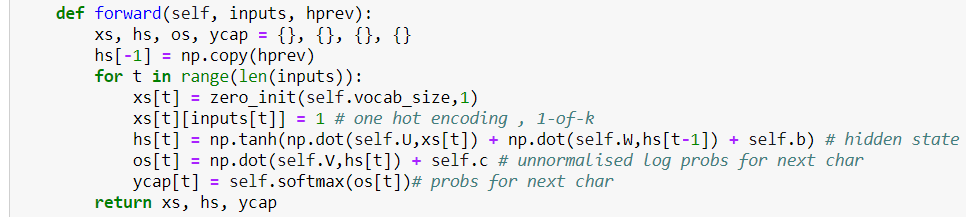## Computing softmax and numerical stability:

Softmax function takes an N-dimensional vector of real numbers and transforms it into a vector of real number in range (0,1) which add upto 1. The mapping is done using the below formula.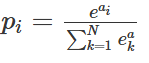The implementation of softmax is: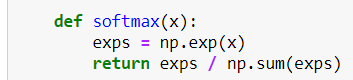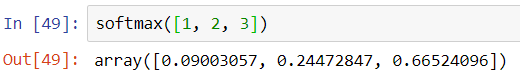Though it looks fine however when we call this softmax with a bigger number like below it gives ‘nan’ values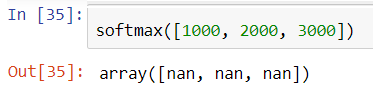The numerical range of the floating-point numbers used by Numpy is limited. For float64, the maximal representable number is on the order of 10³⁰⁸. Exponentiation in the softmax function makes it possible to easily overshoot this number, even for fairly modest-sized inputs. A nice way to avoid this problem is by normalizing the inputs to be not too large or too small. There is a small mathematical trick applied refer here for details. So our softmax looks like: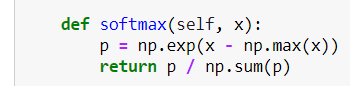## Step 3: Compute Loss

Since we are implementing a text generation model, the next character can be any of the unique characters in our vocabulary. So our loss will be cross-entropy loss. In multi-class classification we take the sum of log loss values for each class prediction in the observation.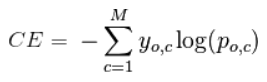• M — number of possible class labels (unique characters in our vocab)
• y — a binary indicator (0 or 1) of whether class label `C`is the correct classification for observation`O`
• p — the model’s predicted probability that observation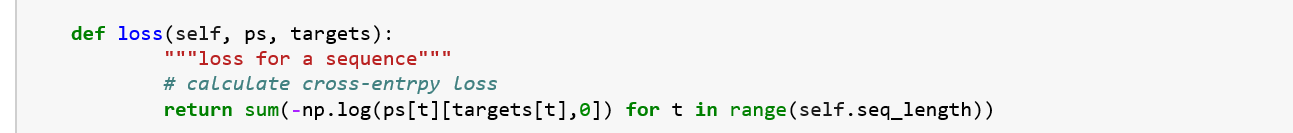## Step 4: Backward pass

If we refer to the BPTT equations, the implementation is as per the equations. Sufficient comments added to understand the code.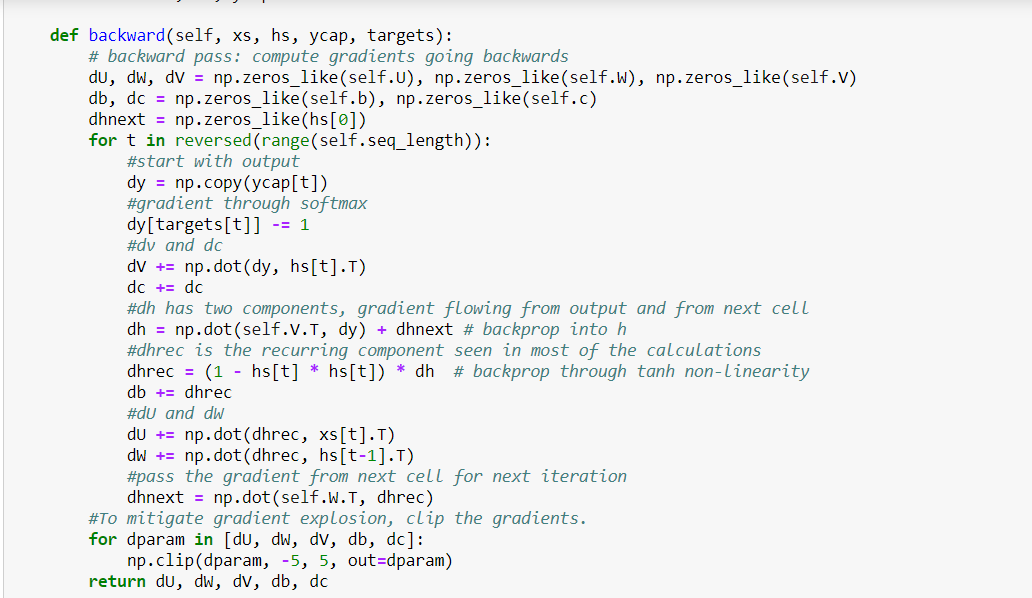## Issues

While in principle the RNN is a simple and powerful model, in practice, it is hard to train properly. Among the main reasons why this model is so unwieldy are the vanishing gradient and exploding gradient problems. While training using BPTT the gradients have to travel from the last cell all the way to the first cell. The product of these gradients can go to zero or increase exponentially. The exploding gradients problem refers to the large increase in the norm of the gradient during training. The vanishing gradients problem refers to the opposite behavior, when long term components go exponentially fast to norm 0, making it impossible for the model to learn correlation between temporally distant events.

Whereas the exploding gradient can be fixed with gradient clipping technique as is used in the example code here, the vanishing gradient issue is still is major concern with an RNN.

This vanishing gradient limitation was overcome by various networks such as long short-term memory (LSTM), gated recurrent units (GRUs), and residual networks (ResNets), where the first two are the most used RNN variants in NLP applications.

## Step 5: Update weights

Using BPTT we calculated the gradient for each parameter of the model. it is now time to update the weights.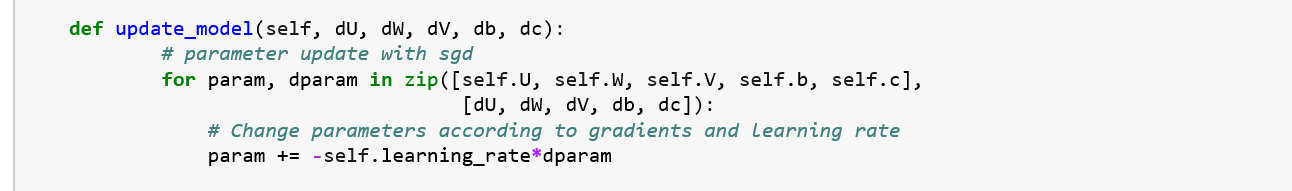In the original implementation by Andrej Karparthy, Adagrad is used for gradient update. Adagrad performs much better than SGD. Please check and compare both.

## Step 6: Repeat steps 2–5

In order for our model to learn from the data and generate text, we need to train it for sometime and check loss after each iteration. If the loss is reducing over a period of time that means our model is learning what is expected of it.

## Generate Text

We train for some time and if all goes well, we should have our model ready to predict some text. Let us see how it works for us.

We will implement a predict method to predict few words like below: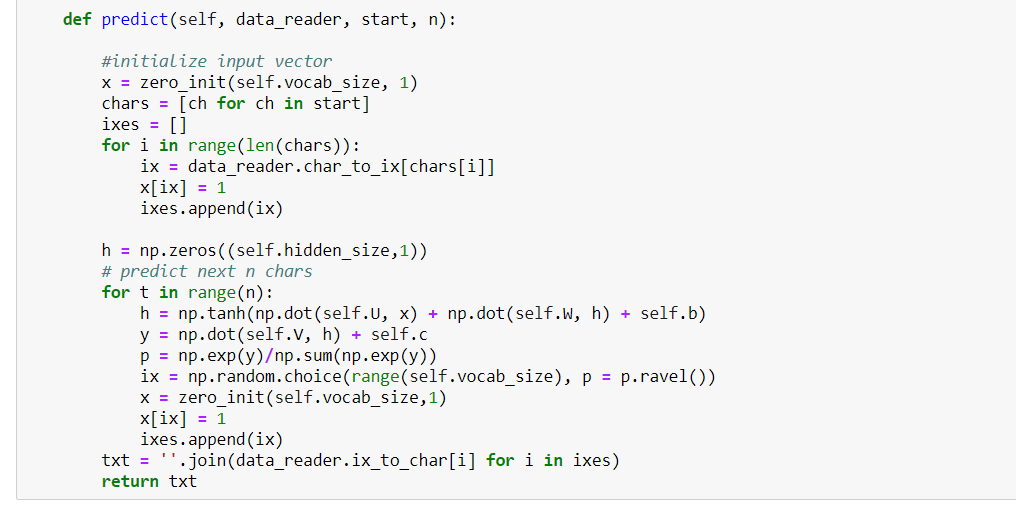Let us see how our RNN is learning after a few epochs of training.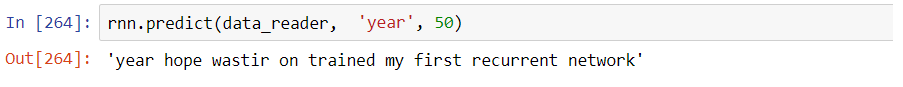The output looks more like real text with word boundaries and some grammar as well. So our baby RNN has staring learning the language and able to predict the next few words.

The implementation presented here just meant to be easy to understand and grasp the concepts. In case you want to play around the model hyper parameters, the notebook is here.

Hope it was useful for you.Thanks for the read.

#python #deep learning

## Buddha Community1619510796

## Lambda, Map, Filter functions in python

Welcome to my Blog, In this article, we will learn python lambda function, Map function, and filter function.

Lambda function in python: Lambda is a one line anonymous function and lambda takes any number of arguments but can only have one expression and python lambda syntax is

Syntax: x = lambda arguments : expression

Now i will show you some python lambda function examples:

#python #anonymous function python #filter function in python #lambda #lambda python 3 #map python #python filter #python filter lambda #python lambda #python lambda examples #python map1624752180

## How to build your own Neural Network from scratch in Python

When discussing neural networks, most beginning textbooks create brain analogies. I can define the new neural networks simply as a mathematical function that translates a certain entry to the desired performance without going into brain analogies.

You may note that the weights W and biases b are the only variables in the equation above affecting the output of a given value. The strength of predictions naturally establishes the correct values for weights and biases. The weight and bias adjustment procedure of the input data is known as neural network training.

#neural-networks #artificial-intelligence #python #programming #technology #how to build your own neural network from scratch in python1626775355

## Why use Python for Software Development

No programming language is pretty much as diverse as Python. It enables building cutting edge applications effortlessly. Developers are as yet investigating the full capability of end-to-end Python development services in various areas.

By areas, we mean FinTech, HealthTech, InsureTech, Cybersecurity, and that's just the beginning. These are New Economy areas, and Python has the ability to serve every one of them. The vast majority of them require massive computational abilities. Python's code is dynamic and powerful - equipped for taking care of the heavy traffic and substantial algorithmic capacities.

Programming advancement is multidimensional today. Endeavor programming requires an intelligent application with AI and ML capacities. Shopper based applications require information examination to convey a superior client experience. Netflix, Trello, and Amazon are genuine instances of such applications. Python assists with building them effortlessly.

## 5 Reasons to Utilize Python for Programming Web Apps

Python can do such numerous things that developers can't discover enough reasons to admire it. Python application development isn't restricted to web and enterprise applications. It is exceptionally adaptable and superb for a wide range of uses.

Robust frameworks

Python is known for its tools and frameworks. There's a structure for everything. Django is helpful for building web applications, venture applications, logical applications, and mathematical processing. Flask is another web improvement framework with no conditions.

Web2Py, CherryPy, and Falcon offer incredible capabilities to customize Python development services. A large portion of them are open-source frameworks that allow quick turn of events.

Simple to read and compose

Python has an improved sentence structure - one that is like the English language. New engineers for Python can undoubtedly understand where they stand in the development process. The simplicity of composing allows quick application building.

The motivation behind building Python, as said by its maker Guido Van Rossum, was to empower even beginner engineers to comprehend the programming language. The simple coding likewise permits developers to roll out speedy improvements without getting confused by pointless subtleties.

Utilized by the best

Alright - Python isn't simply one more programming language. It should have something, which is the reason the business giants use it. Furthermore, that too for different purposes. Developers at Google use Python to assemble framework organization systems, parallel information pusher, code audit, testing and QA, and substantially more. Netflix utilizes Python web development services for its recommendation algorithm and media player.

Massive community support

Python has a steadily developing community that offers enormous help. From amateurs to specialists, there's everybody. There are a lot of instructional exercises, documentation, and guides accessible for Python web development solutions.

Today, numerous universities start with Python, adding to the quantity of individuals in the community. Frequently, Python designers team up on various tasks and help each other with algorithmic, utilitarian, and application critical thinking.

Progressive applications

Python is the greatest supporter of data science, Machine Learning, and Artificial Intelligence at any enterprise software development company. Its utilization cases in cutting edge applications are the most compelling motivation for its prosperity. Python is the second most well known tool after R for data analytics.

The simplicity of getting sorted out, overseeing, and visualizing information through unique libraries makes it ideal for data based applications. TensorFlow for neural networks and OpenCV for computer vision are two of Python's most well known use cases for Machine learning applications.

### Summary

Thinking about the advances in programming and innovation, Python is a YES for an assorted scope of utilizations. Game development, web application development services, GUI advancement, ML and AI improvement, Enterprise and customer applications - every one of them uses Python to its full potential.

The disadvantages of Python web improvement arrangements are regularly disregarded by developers and organizations because of the advantages it gives. They focus on quality over speed and performance over blunders. That is the reason it's a good idea to utilize Python for building the applications of the future.

#python development services #python development company #python app development #python development #python in web development #python software development1596825840

## A Comparative Analysis of Recurrent Neural Networks

Recurrent neural networks, also known as RNNs, are a class of neural networks that allow previous outputs to be used as inputs while having hidden states. RNN models are mostly used in the fields of natural language processing and speech recognition.

The vanishing and exploding gradient phenomena are often encountered in the context of RNNs. The reason why they happen is that it is difficult to capture long term dependencies because of multiplicative gradient that can be exponentially decreasing/increasing with respect to the number of layers.

Gated Recurrent Unit (GRU) and Long Short-Term Memory units (LSTM) deal with the vanishing gradient problem encountered by traditional RNNs, with LSTM being a generalization of GRU.

1D Convolution_ layer_ creates a convolution kernel that is convolved with the layer input over a single spatial (or temporal) dimension to produce a tensor of outputs. It is very effective for deriving features from a fixed-length segment of the overall dataset. A 1D CNN works well for natural language processing (NLP).

## DATASET: IMDb Movie Review

TensorFlow Datasets is a collection of datasets ready to use, with TensorFlow or other Python ML frameworks, such as Jax. All datasets are exposed as `[_tf.data.Datasets_](https://www.tensorflow.org/api_docs/python/tf/data/Dataset)`, enabling easy-to-use and high-performance input pipelines.

“imdb_reviews”

This is a dataset for binary sentiment classification containing substantially more data than previous benchmark datasets. It provides a set of 25,000 highly polar movie reviews for training, and 25,000 for testing.

## Import Libraries

``````import pandas as pd
import numpy as np
import matplotlib.pyplot as plt
import seaborn as sns
%matplotlib inline
``````

## Load the Dataset

``````import tensorflow as tf
import tensorflow_datasets

imdb, info=tensorflow_datasets.load("imdb_reviews", with_info=True, as_supervised=True)
imdb
``````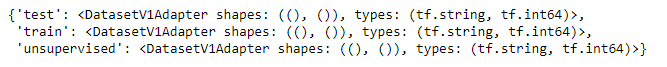``````info
``````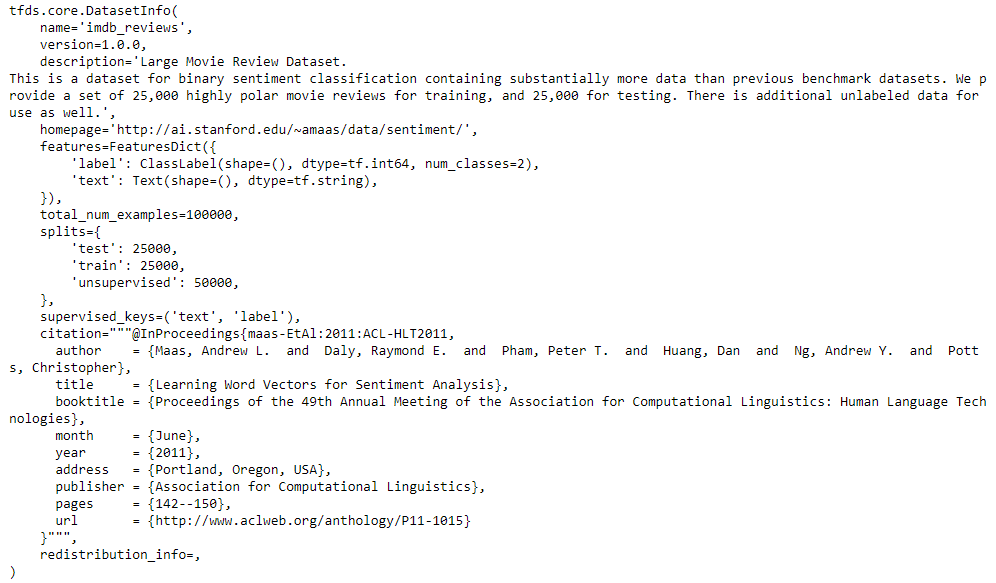## Training and Testing Data

``````train_data, test_data=imdb['train'], imdb['test']

training_sentences=[]
training_label=[]
testing_sentences=[]
testing_label=[]
for s,l in train_data:
training_sentences.append(str(s.numpy()))
training_label.append(l.numpy())
for s,l in test_data:
testing_sentences.append(str(s.numpy()))
testing_label.append(l.numpy())
training_label_final=np.array(training_label)
testing_label_final=np.array(testing_label)
``````

## Tokenization and Padding

``````vocab_size=10000
embedding_dim=16
max_length=120
trunc_type='post'
oov_tok='<oov>'
from tensorflow.keras.preprocessing.text import Tokenizer
from tensorflow.keras.preprocessing.sequence import pad_sequences
tokenizer= Tokenizer(num_words=vocab_size, oov_token=oov_tok)
tokenizer.fit_on_texts(training_sentences)
word_index=tokenizer.word_index
sequences=tokenizer.texts_to_sequences(training_sentences)
testing_sequences=tokenizer.texts_to_sequences(testing_sentences)
from tensorflow.keras.models import Sequential
from tensorflow.keras.layers import Dense, Dropout, Embedding
``````

## Multi-layer Bidirectional LSTM

#imdb #convolutional-network #long-short-term-memory #recurrent-neural-network #gated-recurrent-unit #neural networks1623135499

## No Code introduction to Neural Networks

### The simple architecture explained

Neural networks have been around for a long time, being developed in the 1960s as a way to simulate neural activity for the development of artificial intelligence systems. However, since then they have developed into a useful analytical tool often used in replace of, or in conjunction with, standard statistical models such as regression or classification as they can be used to predict or more a specific output. The main difference, and advantage, in this regard is that neural networks make no initial assumptions as to the form of the relationship or distribution that underlies the data, meaning they can be more flexible and capture non-standard and non-linear relationships between input and output variables, making them incredibly valuable in todays data rich environment.

In this sense, their use has took over the past decade or so, with the fall in costs and increase in ability of general computing power, the rise of large datasets allowing these models to be trained, and the development of frameworks such as TensforFlow and Keras that have allowed people with sufficient hardware (in some cases this is no longer even an requirement through cloud computing), the correct data and an understanding of a given coding language to implement them. This article therefore seeks to be provide a no code introduction to their architecture and how they work so that their implementation and benefits can be better understood.

Firstly, the way these models work is that there is an input layer, one or more hidden layers and an output layer, each of which are connected by layers of synaptic weights¹. The input layer (X) is used to take in scaled values of the input, usually within a standardised range of 0–1. The hidden layers (Z) are then used to define the relationship between the input and output using weights and activation functions. The output layer (Y) then transforms the results from the hidden layers into the predicted values, often also scaled to be within 0–1. The synaptic weights (W) connecting these layers are used in model training to determine the weights assigned to each input and prediction in order to get the best model fit. Visually, this is represented as:

#machine-learning #python #neural-networks #tensorflow #neural-network-algorithm #no code introduction to neural networks